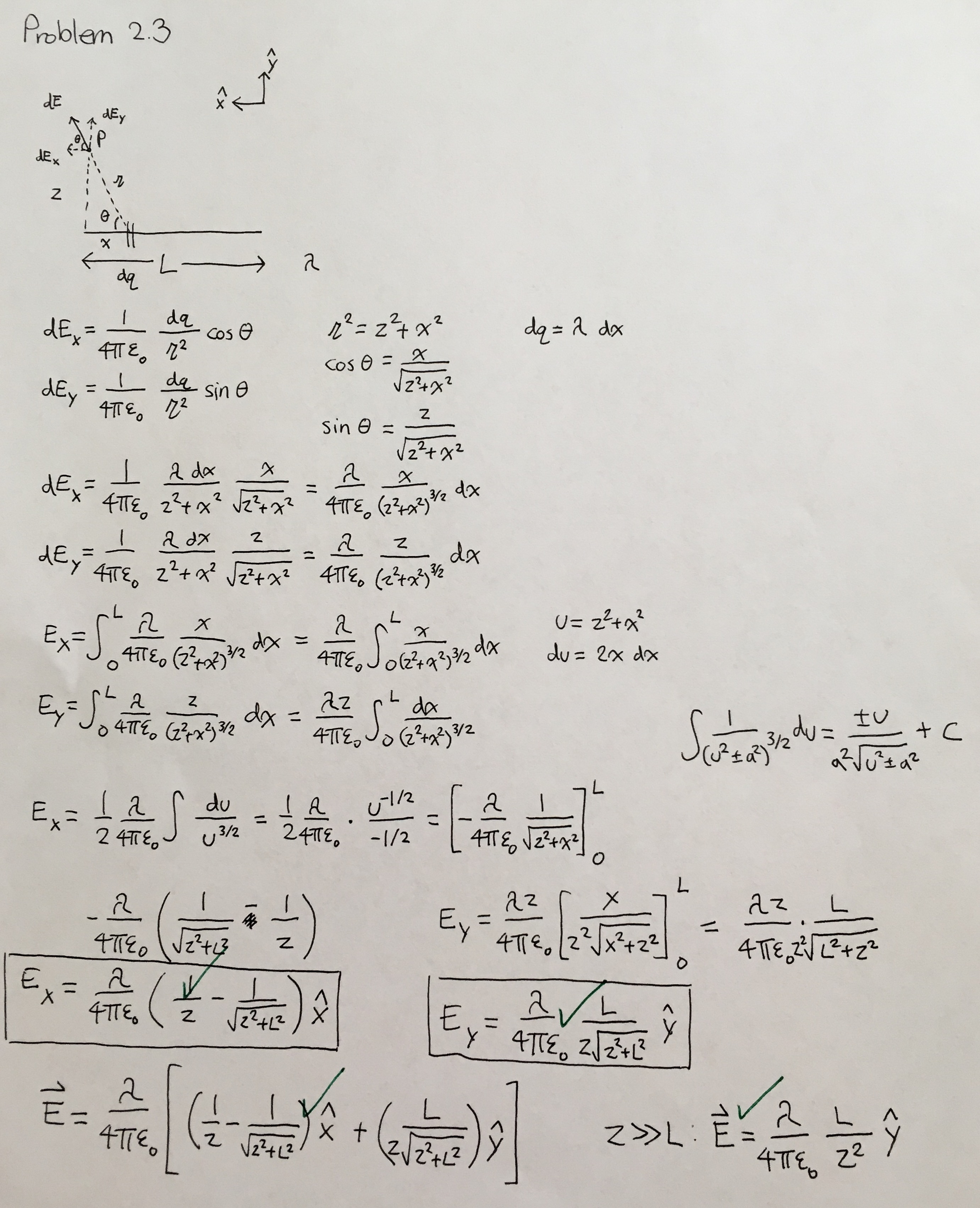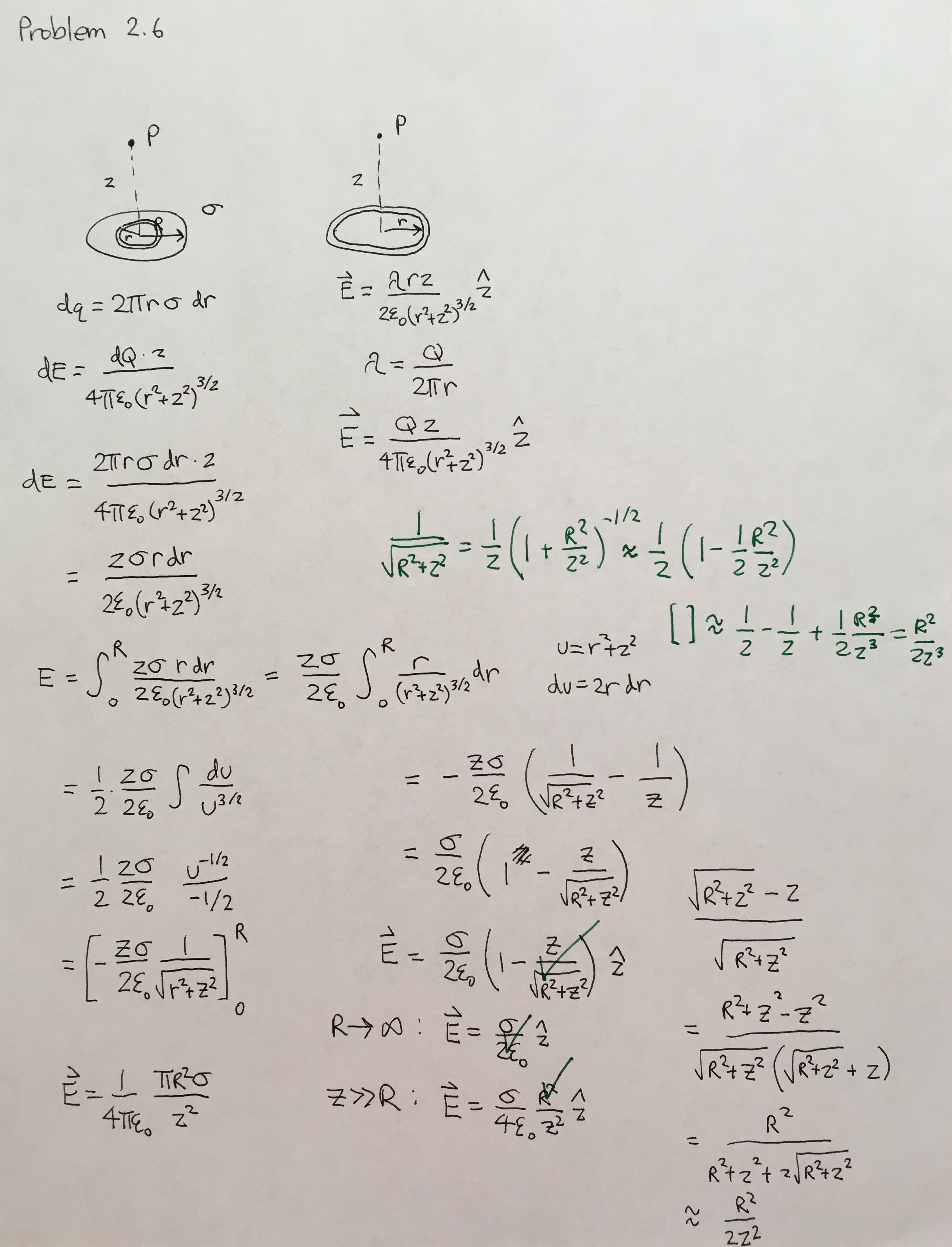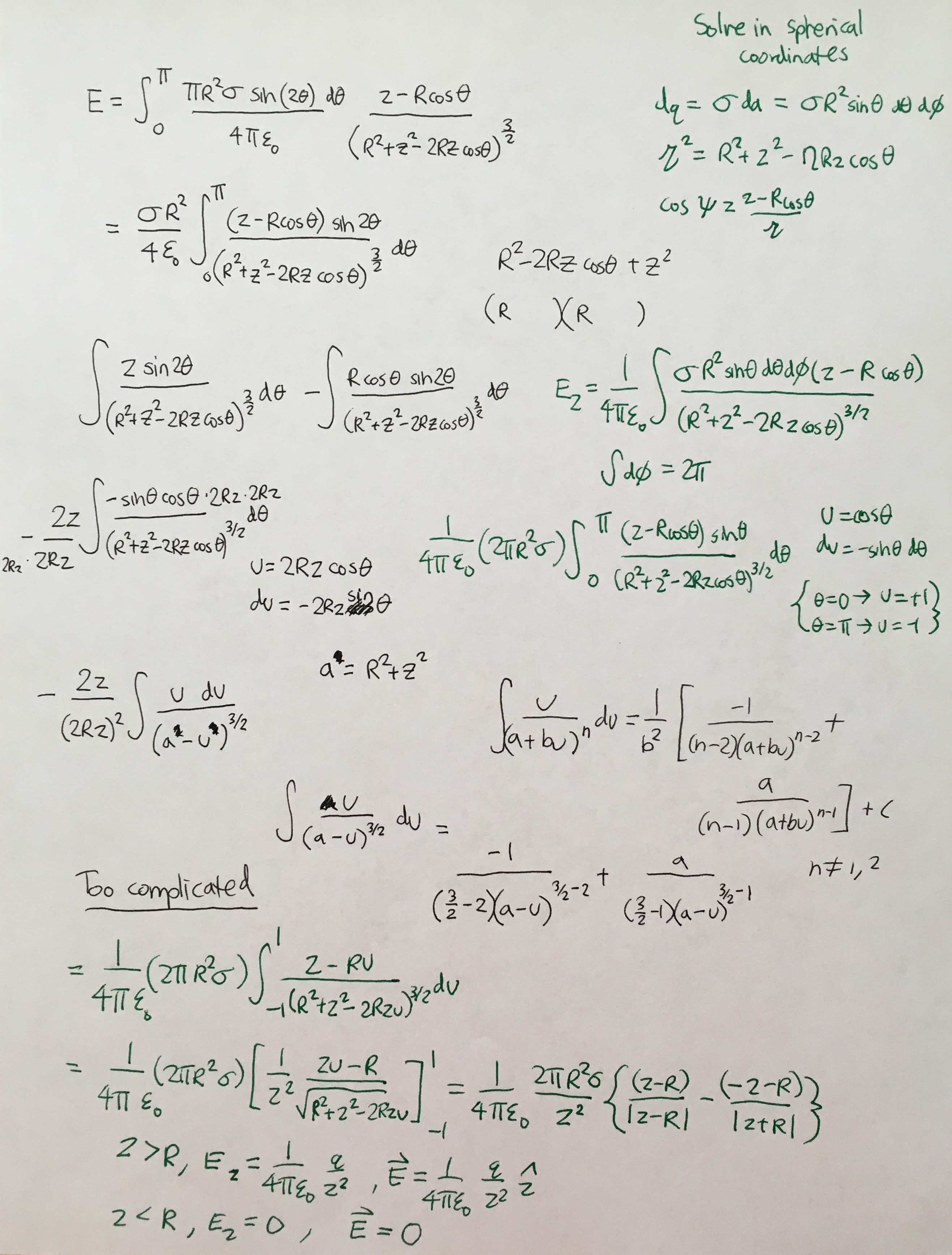# Old

Back

I found some old notes of mine that are solutions to problems found in Griffith’s Electrodynamics and I’ve decided to post them here. My mistakes are included, but I think including them will be instructive for the future student who wishes to avoid the same pitfalls. Note: Corrections are in green.

Problem 2.3
Find the electric field a distance z above one end of a straight line segment of length$L$, which carries a uniform line charge$\lambda$. Check that your formula is consistent with what you would expect for the case$z \gg L$.Problem 2.4
Find the electric field a distance$z$ above the center of a square loop (side$a$) carrying uniform line charge$\lambda$.Problem 2.5
Find the electric field a distance$z$ above the center of a circular loop of radius$r$, which carries a uniform line charge$\lambda$.Problem 2.6
Find the electric field a distance$z$ above the center of a flat circular disk of radius$R$, which carries a uniform surface charge$\sigma$. What does your formula give in the limit$R \rightarrow \infty$? Also check the case$z \gg R$.Problem 2.7
Find the electric field a distance$z$ from the center of a spherical surface of radius$R$, which carries a uniform charge density$\sigma$. Treat the case$zR$ (outside). Express your answers in terms of the total charge$q$ on the sphere.Problem 2.8
Use your result in Problem 2.7 to find the field inside and outside a sphere of radius$R$, which carries a uniform volume charge density$\rho$. Express your answers in terms of the total charge of the sphere,$q$. Draw a graph of$|\vec{E}|$ as a function of the distance from the center.Problem 2.9
Suppose the electric field in some region is found to be$\vec{E}=kr^3\hat{r}$, in spherical coordinates ($k$ is some constant).
a) FInd the charge density$\rho$.
b) Find the total charge contained in a sphere of radius$R$, centered at the origin. (Do it two different ways.)Problem 2.10
A charge$q$ sits at the back corner of a cube. What is the flux of$\vec{E}$ through the shaded side?

Problem 2.11
Use Gauss’s law to find the electric field inside and outside of a spherical shell of radius$R$, which carries a uniform surface charge density$\sigma$.Problem 2.12
Use Gauss’s law to find the electric field inside a uniformly charged sphere (charge density$\rho$).

Problem 2.13
Find the electric field a distance$s$ from an infinitely long straight wire, which carries a uniform line charge$\lambda$.

Problem 2.14
Find the electric field inside a sphere which carries a charge density proportional to the distance from the origin,$\rho=kr$, for some constant$k$.